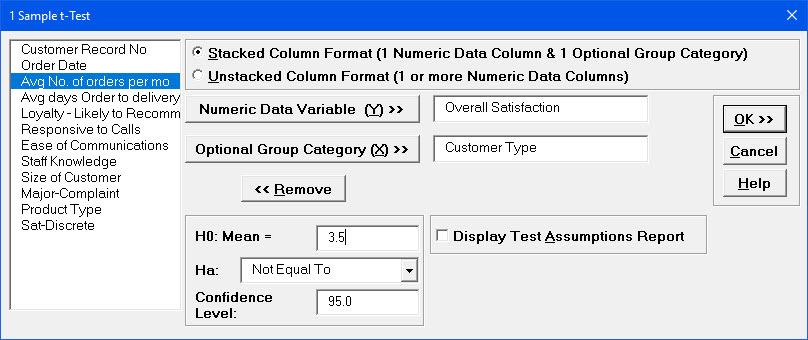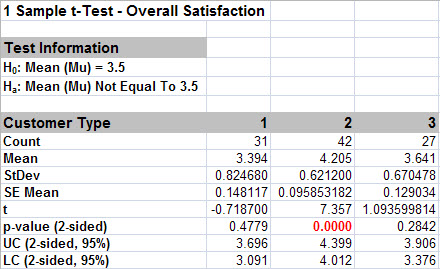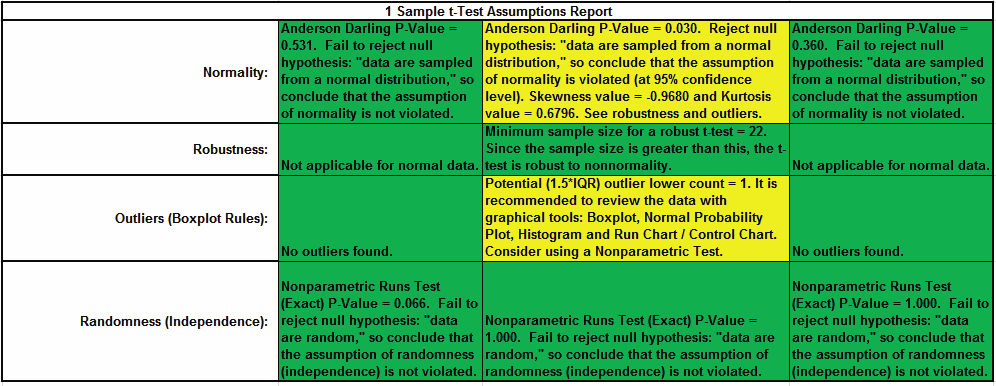Include Top

# How Do I Perform a One Sample t-Test in Excel Using SigmaXL?

1. Open Customer Data.xlsx, select Sheet 1 tab (or press F4 to activate last worksheet). Click SigmaXL > Statistical Tools > 1 Sample t-Test & Confidence Intervals. If necessary, check Use Entire Data Table, click Next.

2. Ensure that Stacked Column Format is selected. Select Overall Satisfaction, click Numeric Data Variable (Y) >>, select Customer Type, click Optional Group Category (X) >>.

3.  Historically, our average customer satisfaction score has been 3.5. We would like to see if this has changed, with the results grouped by customer type. Null Hypothesis H0: μ=3.5; Alternative Hypothesis Ha: μ≠3.5

4. Enter 3.5 for the Null Hypothesis H0: Mean value. Keep Ha as Not Equal To.

5.6. Click OK. Results:

7.8. Note the p-values. Customer Type 2 shows a significant change (increase) in Satisfaction Mean (p-value < .05), whereas Customer Types 1 and 3 show no change (p-value ≥ .05). Also note the confidence intervals around each mean match the results from Descriptive Statistics.

9. In the Measure Phase we determined that Overall Satisfaction for Customer Type 2 has non-normal data but this does not imply that the p-value for the 1 Sample t-test is wrong. The Central Limit Theorem applies here: the distribution of averages tends to be normal, even if the individual observations are not-normal. With a sample size of 42, the t-test is fairly robust against skewed data.

10. 1 Sample t-Test Assumptions Report:This is a text report with color highlight: Green (OK), Yellow (Warning) and Red (Serious Violation).
Each sample is tested for Normality using the Anderson Darling test. If not normal, the minimum sample size for robustness of the 1 sample t-Test is determined utilizing Monte Carlo regression equations (see Basic Statistical Templates – Minimum Sample Size for Robust t-Tests and ANOVA). If the sample size is inadequate, a warning is given and a suitable Nonparametric Test is recommended (Wilcoxon if symmetric, Sign Test if not symmetric).

Each sample is tested for Outliers defined as: Potential: Tukey's Boxplot (> Q3 + 1.5*IQR or < Q1 – 1.5*IQR); Likely: Tukey's Boxplot 2*IQR; Extreme: Tukey's Boxplot 3*IQR. If outliers are present, a warning is given and recommendation to review the data with a Boxplot and Normal Probability Plot. Here we have a potential outlier for Customer Type 2.

Tip: If the removal of outlier(s) result in an Anderson Darling P-Value that is > 0.1, a notice is given that excluding the outlier(s), the sample data are inherently normal.

Each sample is also tested for Randomness using the Exact Nonparametric Runs Test. If the sample data is not random, a warning is given and recommendation to review the data with a Run Chart.

# Web Demos

Our CTO and Co-Founder, John Noguera, regularly hosts free Web Demos featuring SigmaXL and DiscoverSim